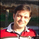5091 visningar
Ok, Here we go - Version 1 of TTM Reversion Band goes live.

For the amount of information this has, the price tag is not hefty.

John's RTM Chapter Synopsis - http://www.tradethemarkets.com/public/ma...

What did I modify?

1. Automated the Daily + and Intraday settings. So Switching to intraday will change the setting automatically.

2. Added text callouts for RTM condition
a. John Carter's Indicator

Future Improvement Planned.

1. Will Convert this into a system based on John's Indicator from TS .

2. Custom Options for Slope and MA setting for Trade filter.
```// Created by UCSgears
// Adopted from TTM Reversion Bands

study(title="UCS_TTM - Reversion Bands", shorttitle="UCS_TTM-RTM", overlay=true)

// Defining Timeframe and Parameters
a = isdwm ? 1 : 0
b = isintraday ? 1 : 0

// TTM - Revertion to the Mean Band - INPUTS
z = input(0, title = "Offset")
usesl = input(true, title = "RTM against Slope (UCSgears Addition)", type=bool)
length = a == 1 ? 13 : 25
atrlen = a == 1 ? 13 : 25
mult = a == 1 ? 1.5 : 2.5
range =  tr

// Calculations
ma = ema(close, length)
rangema = ema(range, atrlen)
upper = ma + rangema * mult
lower = ma - rangema * mult

// All Plots
plot(upper, color=purple, title="Upper Channel", offset = z)
plot(ma, color=red, title="Middle Line", offset = z)
plot(lower, color=purple, title="Lower Channel", offset = z)

//Slope Definition
slope = (ma - ma)/5

sh = highest(slope, 250)
sl = lowest(slope, 250)
sm = 0 /////////////////////////////////////////////////////////////////////////////////////////////////(sh+sl)/2

pup = slope > sm ? 1 : 0
ndn = slope < sm ? 1 : 0

plotshape(usesl ? pup : na, style = shape.triangleup, color = green, location = location.bottom, title = "Positive Slope", transp = 0)
plotshape(usesl ? ndn : na, style = shape.triangledown, color = red, location = location.top, title = "Negative Slope", transp = 0)

// Condition for Dots
dota = (high > upper) and (high < upper) ? upper : na
dotb = (low  < lower) and (low  > lower) ? lower : na
dotbsp = pup == 1 ? dotb : na
dotasn = ndn == 1 ? dota : na

plotshape(usesl ? dotasn : dota, style = shape.circle, location = location.abovebar, color = fuchsia, title = "RTM UP", text = "RTM - UP \n SHORT", textcolor = fuchsia, transp = 0)
plotshape(usesl ? dotbsp : dotb, style = shape.circle, location = location.belowbar, color = blue, title = "RTM Down", text = "RTM - DOWN \n LONG", textcolor = blue, transp = 0)```

## KommentarerI asked a question on another post ... the one with the one with the top and bottom candle identifier script. Do you mind having a look?
Svara